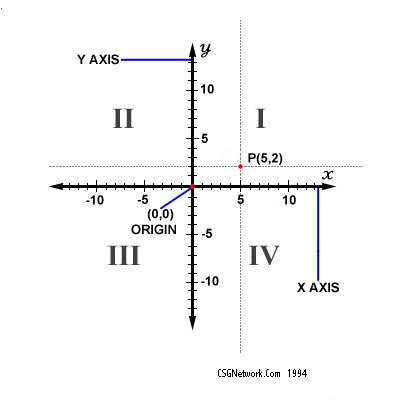# Cartesian Coordinate Distance Calculator

In our example, the arrows on the axes indicate that they extend infinitely in that direction. The intersection of the two x-y axes creates four quadrants indicated by the Roman numerals I, II, III, and IV. By definition, the quadrants are labeled counter-clockwise starting from the upper right quadrant. In quadrant I the values are both positive, (x,y), in quadrant II, they are mixed, (-x,y), in quadrant III, they are both negative, (-x,-y), and in quadrant IV, they are mixed, (x,-y). Point P is in quadrant I, at coordinates (5,2).

Enter a coordinate pair for each the starting and ending points according to the Cartesian coordinate system. Then click on "Calculate". The calculator will determine the straight line distance between the two points as well as present the coordinates of each point as entered in the standard designation.

 Unit Number Required Data Entry xA abscissa yA ordinate xB abscissa yB ordinate Calculated Results Point A Coordinates Point B Coordinates Resulting Distance Rounded Resulting Distance
Updated 8.13.11Leave us a question or comment on FacebookSearch or Browse Our Site